# By Velar H Elias MEASUREMENT INTRODUCTION Measurement measurement

• Slides: 40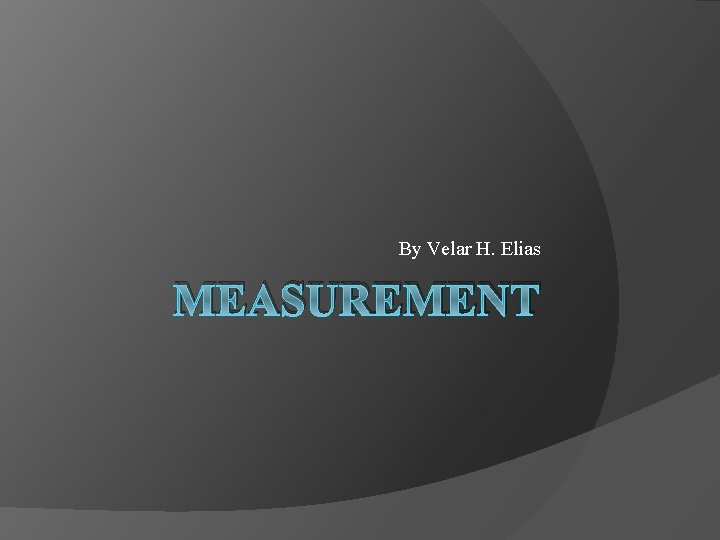By Velar H. Elias MEASUREMENTINTRODUCTION � Measurement: measurement means, to monitor a process or a operation and using an instrument, express the parameter, quantity or a variable in terms of meaningful numbers. � Measurement of a given parameter or quantity is the act or result of a quantitative comparison between a predefined standard an unknown quantity to be measured.Evolution of Instruments I Mechanical b) Electrical c) Electronic Instruments a) A. Mechanical: these instruments are very reliable for static and stable conditions. But their disadvantage is that they are unable to respond rapidly to measurements of dynamic and transient conditions.Evolution of Instruments II B. Electrical: it is faster than mechanical, indicating the output are rapid than mechanical methods. But it depends on the mechanical movement of the meters. The response is between 0. 5 to 24 seconds. C. Electronic: it is more reliable then other system. It uses semiconductor devices and weak signal can also be detected.Advantages of Electronic Measurement � � � Most of the quantities can be converted by transducers into the electrical or electronic signals. Electronic signals can be amplified, filtered, multiplexed, sampled and measured. Measured signals can be transmitted over long distance through cables or radio links, without any loss of information. Many measurements can be done simultaneously or in rapid succession. Electronic circuits can measure the events of very short duration. Higher sensitivity, low power consumption and a higher degree of reliability are the important features.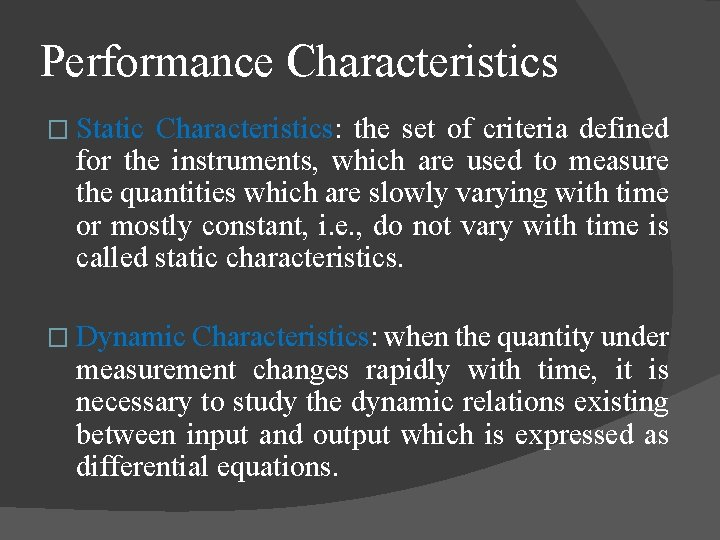Performance Characteristics � Static Characteristics: the set of criteria defined for the instruments, which are used to measure the quantities which are slowly varying with time or mostly constant, i. e. , do not vary with time is called static characteristics. � Dynamic Characteristics: when the quantity under measurement changes rapidly with time, it is necessary to study the dynamic relations existing between input and output which is expressed as differential equations.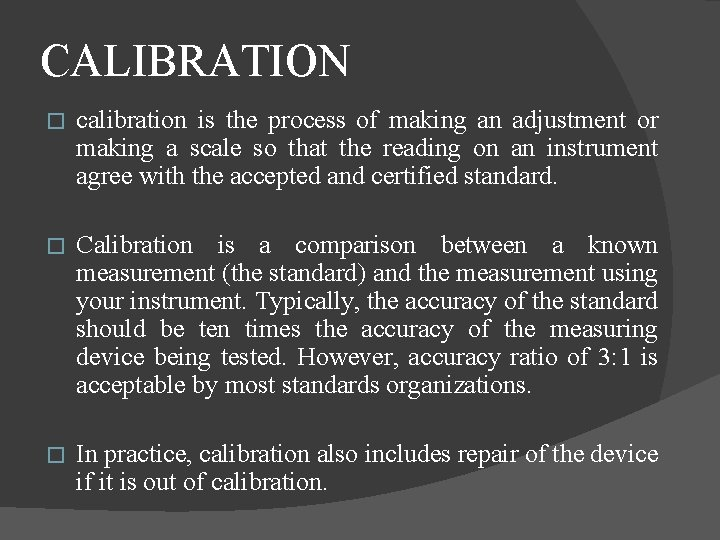CALIBRATION � calibration is the process of making an adjustment or making a scale so that the reading on an instrument agree with the accepted and certified standard. � Calibration is a comparison between a known measurement (the standard) and the measurement using your instrument. Typically, the accuracy of the standard should be ten times the accuracy of the measuring device being tested. However, accuracy ratio of 3: 1 is acceptable by most standards organizations. � In practice, calibration also includes repair of the device if it is out of calibration.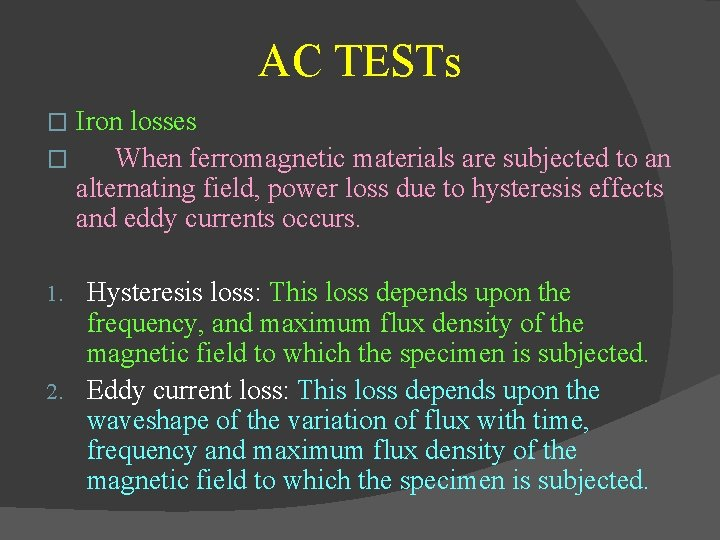AC TESTs Iron losses � When ferromagnetic materials are subjected to an alternating field, power loss due to hysteresis effects and eddy currents occurs. � Hysteresis loss: This loss depends upon the frequency, and maximum flux density of the magnetic field to which the specimen is subjected. 2. Eddy current loss: This loss depends upon the waveshape of the variation of flux with time, frequency and maximum flux density of the magnetic field to which the specimen is subjected. 1.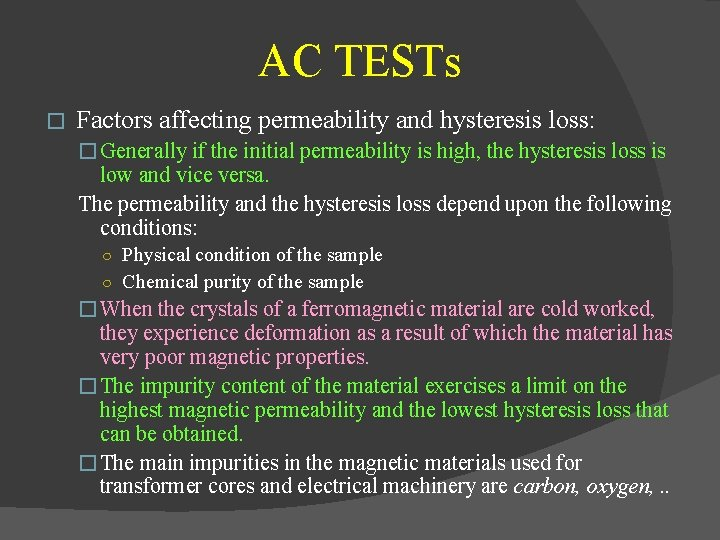AC TESTs � Factors affecting permeability and hysteresis loss: � Generally if the initial permeability is high, the hysteresis loss is low and vice versa. The permeability and the hysteresis loss depend upon the following conditions: ○ Physical condition of the sample ○ Chemical purity of the sample � When the crystals of a ferromagnetic material are cold worked, they experience deformation as a result of which the material has very poor magnetic properties. � The impurity content of the material exercises a limit on the highest magnetic permeability and the lowest hysteresis loss that can be obtained. � The main impurities in the magnetic materials used for transformer cores and electrical machinery are carbon, oxygen, . .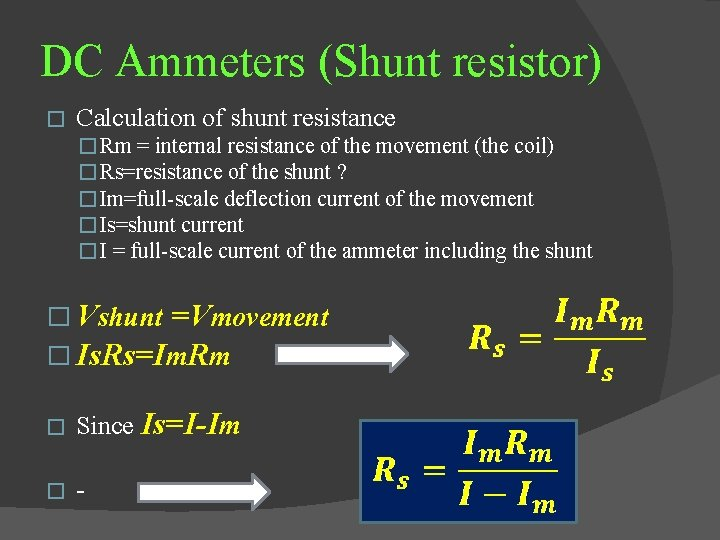DC Ammeters (Shunt resistor) � Calculation of shunt resistance � Rm = internal resistance of the movement (the coil) � Rs=resistance of the shunt ? � Im=full scale deflection current of the movement � Is=shunt current � I = full scale current of the ammeter including the shunt � Vshunt =Vmovement � Is. Rs=Im. Rm � Since Is=I-Im �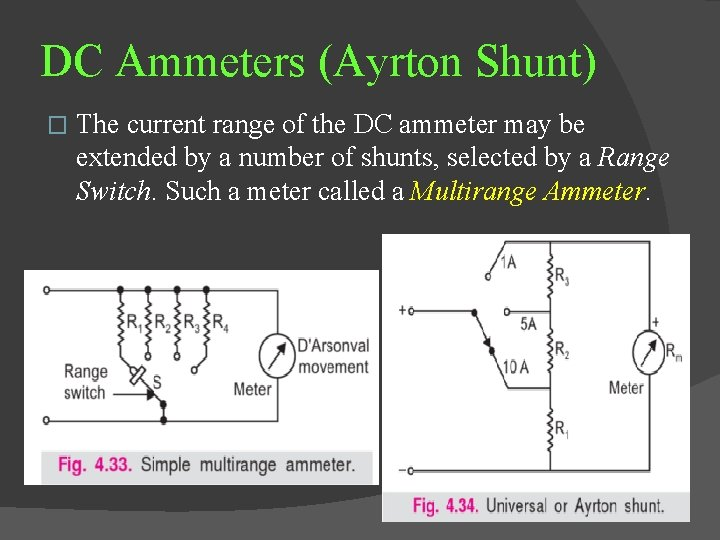DC Ammeters (Ayrton Shunt) � The current range of the DC ammeter may be extended by a number of shunts, selected by a Range Switch. Such a meter called a Multirange Ammeter.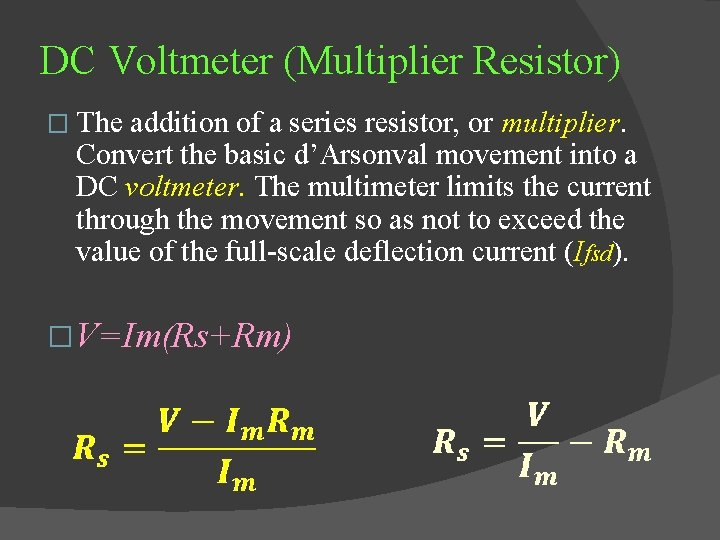DC Voltmeter (Multiplier Resistor) � The addition of a series resistor, or multiplier. Convert the basic d’Arsonval movement into a DC voltmeter. The multimeter limits the current through the movement so as not to exceed the value of the full scale deflection current (Ifsd). � V=Im(Rs+Rm)Shunt type OhmmeterAC Indicating Instruments � The d’Arsonval movement responds to the average or DC value of the current through the moving coil. If the movement current carries an AC with positive and negative half cycles, the driving torque would be in one direction for the positive alternation and in the other direction for the negative alternation. For very low frequency, the pointer would swing around the zero point on the meter scale. � For high frequency, the inertia of the coil is so great the pointer cannot follow the rapid reversals of the driving torque and hovers around the zero mark, vibrating slightly. � � To measure AC on a d’Arsonval movement some means must be devised to obtain a unidirectional torque. First is rectification of the AC, and second is using heating effect of the AC to produce an indication of its magnitude.Rectifier –Type instruments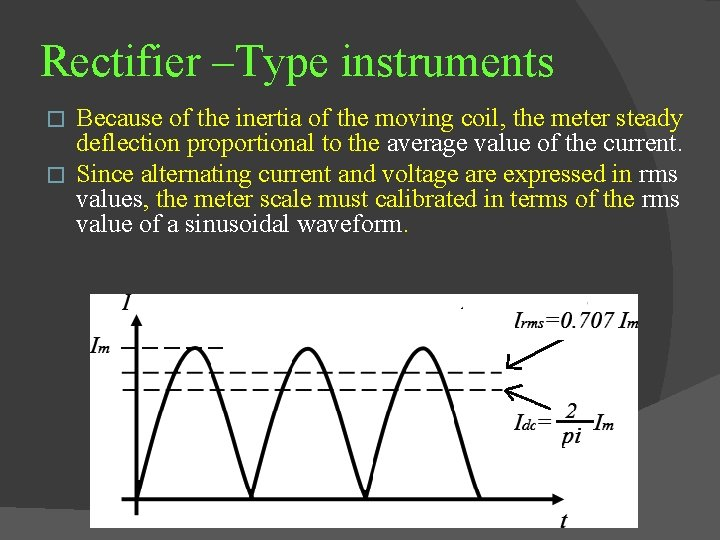Rectifier –Type instruments Because of the inertia of the moving coil, the meter steady deflection proportional to the average value of the current. � Since alternating current and voltage are expressed in rms values, the meter scale must calibrated in terms of the rms value of a sinusoidal waveform. �Typical Multimeter circuits Without D 2 With D 2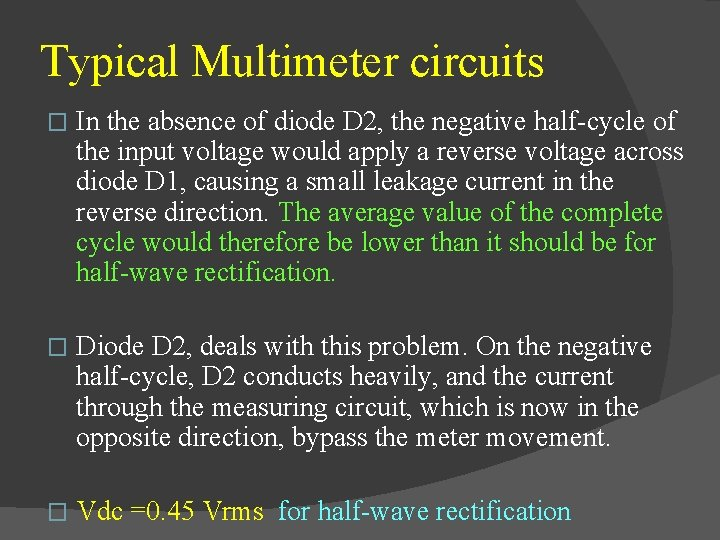Typical Multimeter circuits � In the absence of diode D 2, the negative half cycle of the input voltage would apply a reverse voltage across diode D 1, causing a small leakage current in the reverse direction. The average value of the complete cycle would therefore be lower than it should be for half wave rectification. � Diode D 2, deals with this problem. On the negative half cycle, D 2 conducts heavily, and the current through the measuring circuit, which is now in the opposite direction, bypass the meter movement. � Vdc =0. 45 Vrms for half wave rectification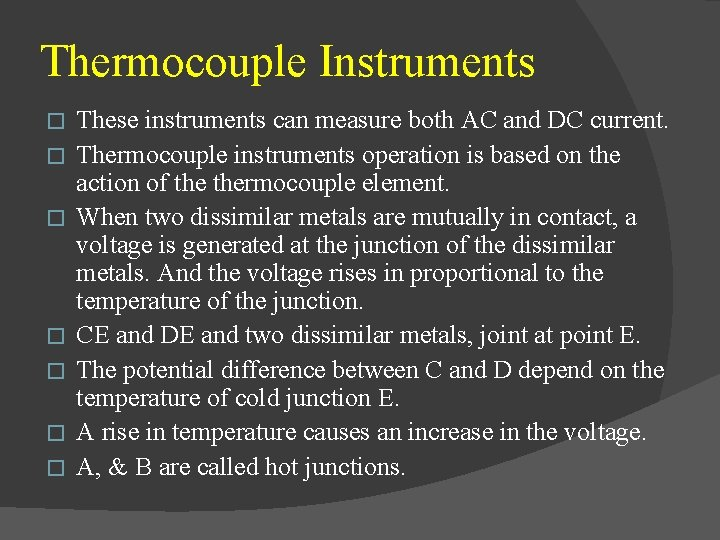Thermocouple Instruments � � � � These instruments can measure both AC and DC current. Thermocouple instruments operation is based on the action of thermocouple element. When two dissimilar metals are mutually in contact, a voltage is generated at the junction of the dissimilar metals. And the voltage rises in proportional to the temperature of the junction. CE and DE and two dissimilar metals, joint at point E. The potential difference between C and D depend on the temperature of cold junction E. A rise in temperature causes an increase in the voltage. A, & B are called hot junctions.Electrodynamometer � � � Electrodynamometer is the one of the most important AC movement, used in accurate AC voltmeters and ammeters, not only at the powerline frequency but also in the lower audio-frequency range. With some slight modification, can be used as a wattmeter, a VARmeter, a power factor meter, or a frequency meter. May also serve as a transfer instrument, because it can be calibrated on DC and then used directly on AC. The d’Arsonval movement uses a permanent magnet to provide the magnetic field in which the movable coil rotates. The electrodynamometer uses the current under measurement to produce the necessary field flux.Electrodynamometer A fixed coil, split into two equal halves, providing the magnetic field in which the movable coil rotates. � The two halves are connected in series with moving coil and are fed by current under measurement. � Ø Like d’Arsonval movement, its rotation is controlled by a springs. Ø And damping is provided by aluminum air vanes, moving in sectorshaped chambers.Electrodynamometer Torque = B*A*I*N � 1 (T α A) and (T α N) “torque depend on design” � But A and N are constants � 2 (T α I) “directly depend on current” � 3 but (B α I) “because the flux is generated by current” � � 4 then ( T α I² )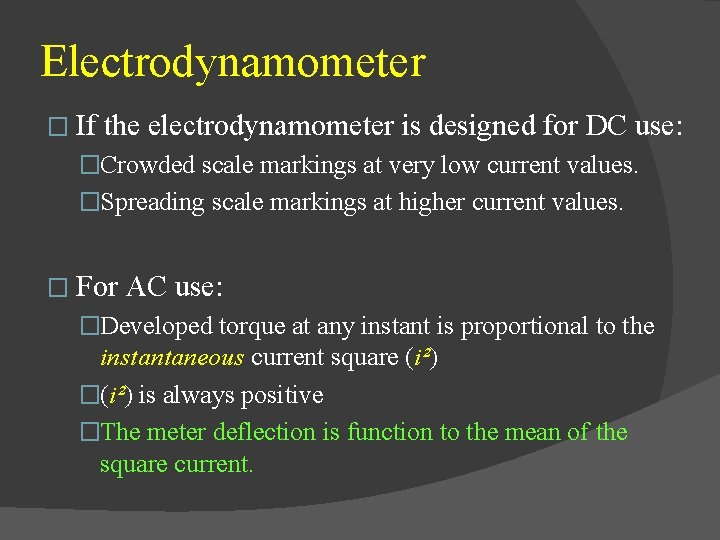Electrodynamometer � If the electrodynamometer is designed for DC use: �Crowded scale markings at very low current values. �Spreading scale markings at higher current values. � For AC use: �Developed torque at any instant is proportional to the instantaneous current square (i²) �(i²) is always positive �The meter deflection is function to the mean of the square current.Electrodynamometer � The scale of the electrodynamometer is usually calibrated in terms of the square root of the average current square: � Therefore, the meter reads the rms or effective value of the AC. � The transfer properties of the electrodynamometer becomes apparent when we compare the effective value of alternative current and direct current in terms of their heating effect or transfer of power.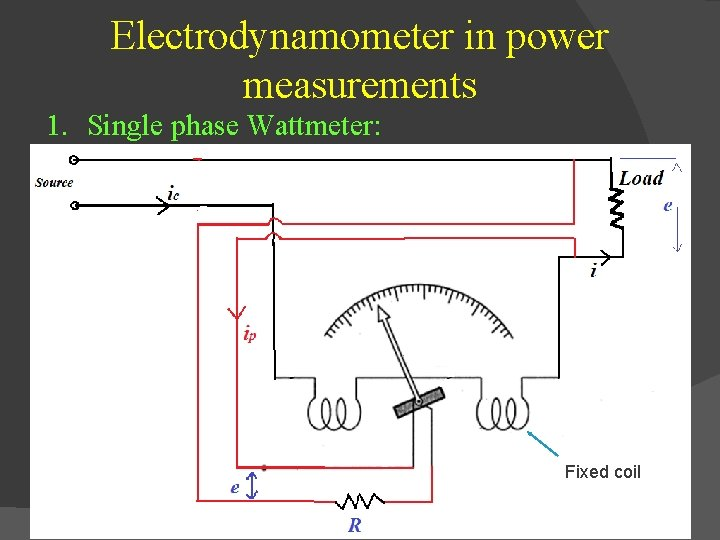Electrodynamometer in power measurements 1. Single phase Wattmeter: Fixed coil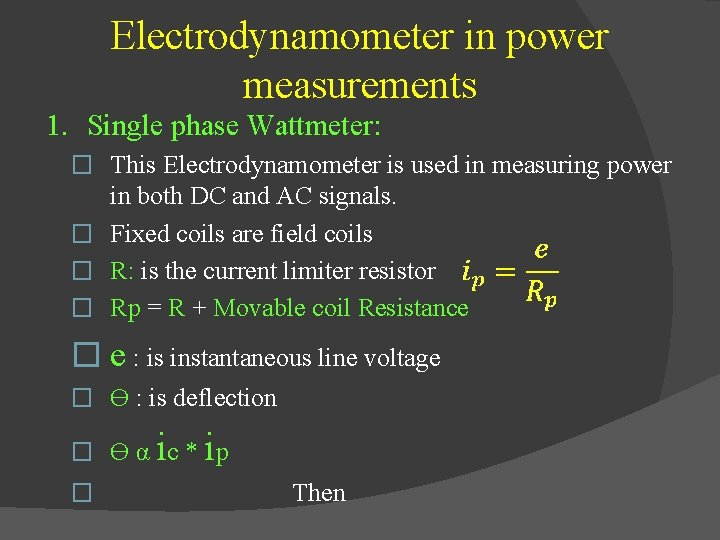Electrodynamometer in power measurements 1. Single phase Wattmeter: � This Electrodynamometer is used in measuring power in both DC and AC signals. � Fixed coils are field coils � R: is the current limiter resistor � Rp = R + Movable coil Resistance � e : is instantaneous line voltage � ϴ : is deflection i i � ϴ α c * p � Then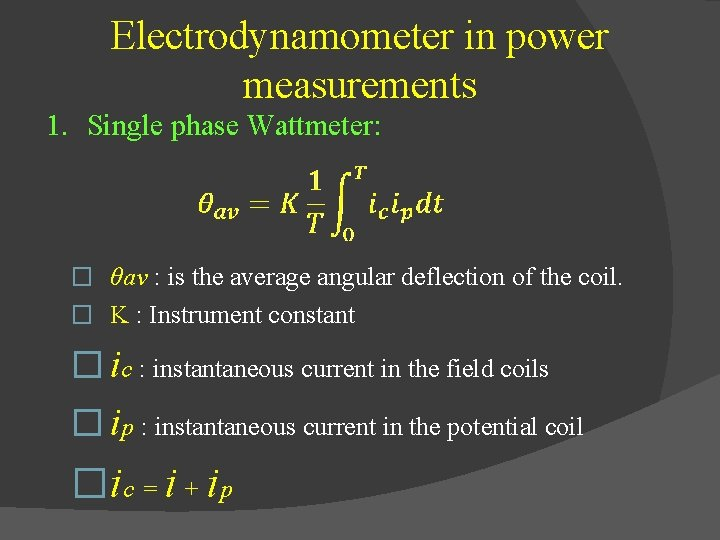Electrodynamometer in power measurements 1. Single phase Wattmeter: � θav : is the average angular deflection of the coil. � K : Instrument constant � ic : instantaneous current in the field coils � ip : instantaneous current in the potential coil �ic = i + ipElectrodynamometer in power measurements 2. Power factor meters: � PF=cos θ � The power factor meter is the cosine of the phase angle between current and voltage. � The instrument is basically an electrodynamometer movement, where the moving element consists of two coils, mounted on the same shaft but at right angles to each other. Lag Lead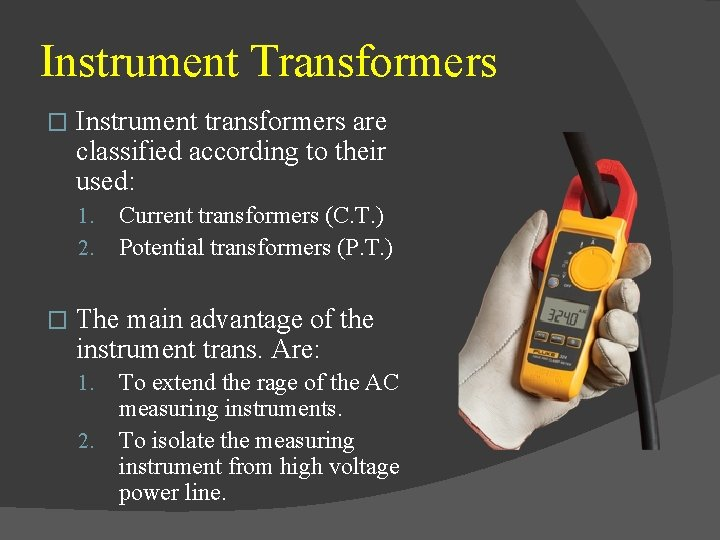Instrument Transformers � Instrument transformers are classified according to their used: 1. 2. � Current transformers (C. T. ) Potential transformers (P. T. ) The main advantage of the instrument trans. Are: 1. 2. To extend the rage of the AC measuring instruments. To isolate the measuring instrument from high voltage power line.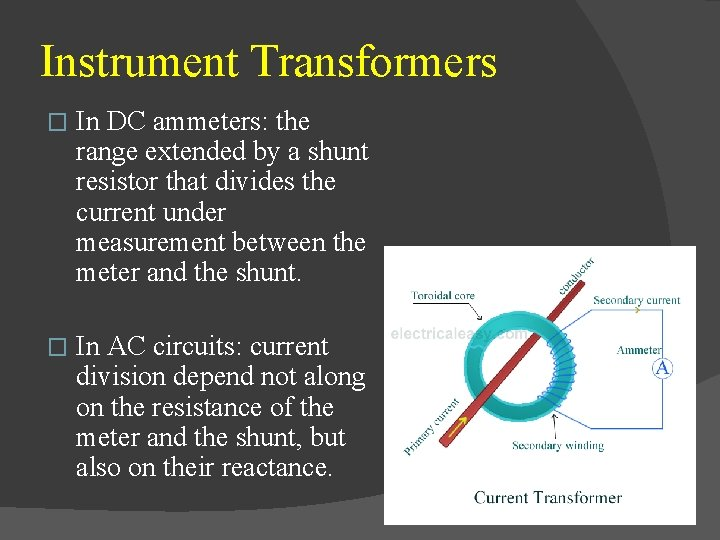Instrument Transformers � In DC ammeters: the range extended by a shunt resistor that divides the current under measurement between the meter and the shunt. � In AC circuits: current division depend not along on the resistance of the meter and the shunt, but also on their reactance.Instrument Transformers � C. T. �Some times has a primary winding �But always has a Secondary winding. �If there is a primary winding, it has a small number of terns. In most cases, the primary winding consist of one turn or a single conductor in series with the load whose current is to measured. �Secondary winding has a larger number of terns and is connected to a current meter. � P. T. �The PT must satisfy certain design requirements that include accuracy of the turns ratio, small leakage reactance, small magnetizing current, and minimal voltage drop.OSCILLOSCOPES � The heart of oscilloscope is the Cathode Ray tube, which: � generate the electron beam, �accelerates the beam to a high speed, �deflect the beam to create the image, �contains the phosphor screen where the electron beam eventually becomes visible.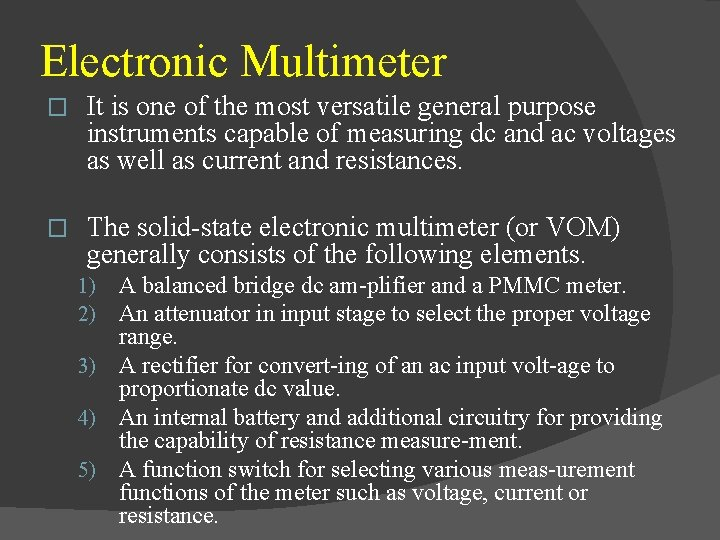Electronic Multimeter � It is one of the most versatile general purpose instruments capable of measuring dc and ac voltages as well as current and resistances. � The solid state electronic multimeter (or VOM) generally consists of the following elements. A balanced bridge dc am plifier and a PMMC meter. An attenuator in input stage to select the proper voltage range. 3) A rectifier for convert ing of an ac input volt age to proportionate dc value. 4) An internal battery and additional circuitry for providing the capability of resistance measure ment. 5) A function switch for selecting various meas urement functions of the meter such as voltage, current or resistance. 1) 2)Digital Voltmeters DVMs can be classified to the following catagories � 1. 2. 3. 4. Ramp type DVM Integrating DVM Continuous balance DVM Successive approximation DVM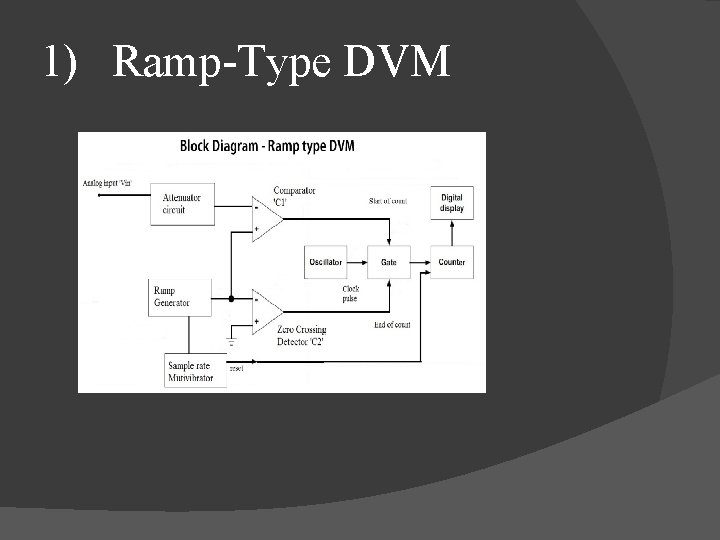1) Ramp Type DVM1) Ramp Type DVM � At the start of the measurement cycle, a ramp voltage is initiated; this voltage can be positive going or negative going. � The negative going shows in previous figure, is continuously compared with the unknown input voltage. � At the instant the ramp voltage equals to the unknown voltage, a coincidence circuit, or comparator, generates a pulse which opens a gate.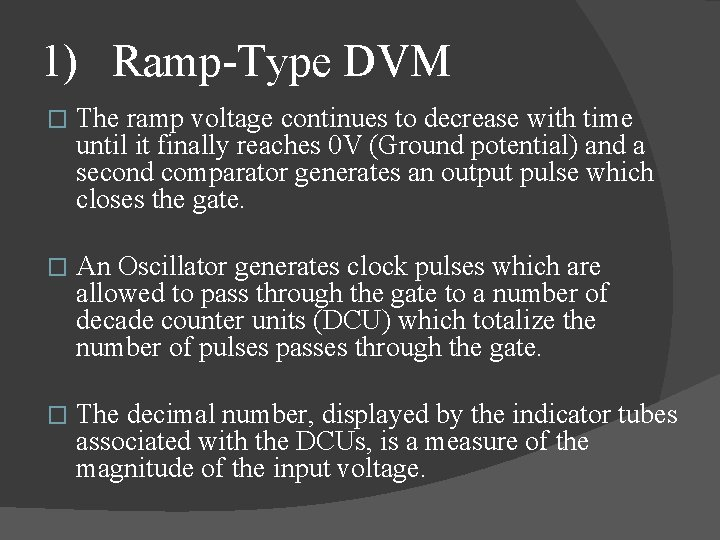1) Ramp Type DVM � The ramp voltage continues to decrease with time until it finally reaches 0 V (Ground potential) and a second comparator generates an output pulse which closes the gate. � An Oscillator generates clock pulses which are allowed to pass through the gate to a number of decade counter units (DCU) which totalize the number of pulses passes through the gate. � The decimal number, displayed by the indicator tubes associated with the DCUs, is a measure of the magnitude of the input voltage.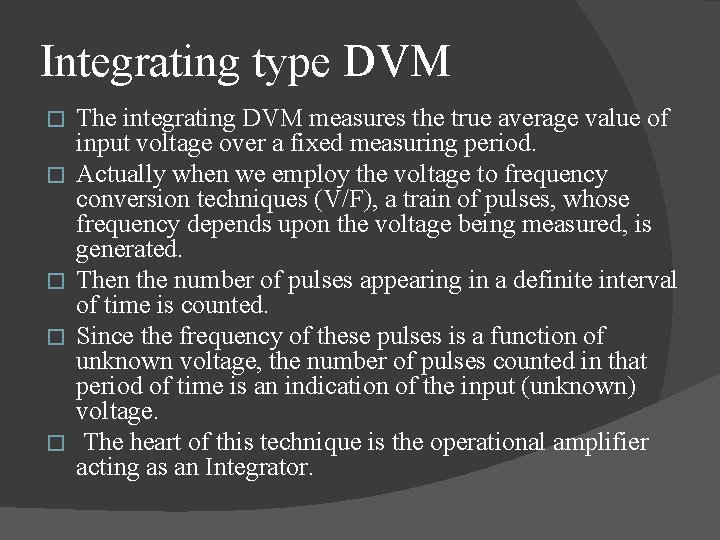Integrating type DVM � � � The integrating DVM measures the true average value of input voltage over a fixed measuring period. Actually when we employ the voltage to frequency conversion techniques (V/F), a train of pulses, whose frequency depends upon the voltage being measured, is generated. Then the number of pulses appearing in a definite interval of time is counted. Since the frequency of these pulses is a function of unknown voltage, the number of pulses counted in that period of time is an indication of the input (unknown) voltage. The heart of this technique is the operational amplifier acting as an Integrator.Staircase Ramp DVM � This DVM makes voltage measurements by comparing the input voltage to an internally generated staircase ramp voltage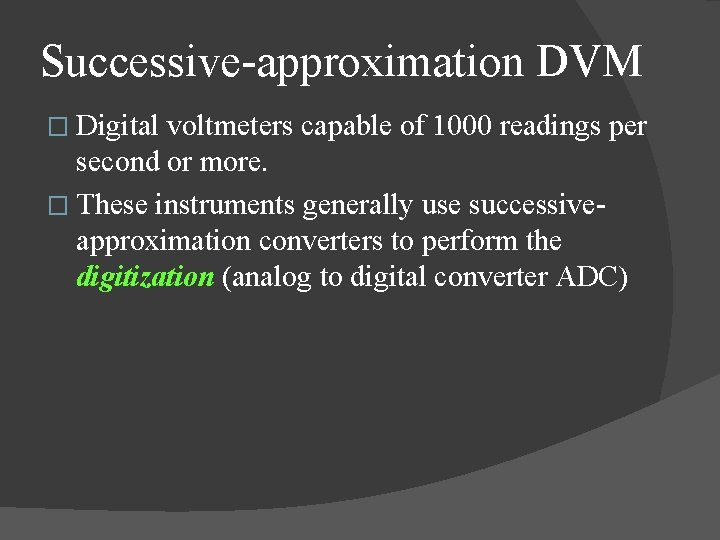Successive approximation DVM � Digital voltmeters capable of 1000 readings per second or more. � These instruments generally use successive approximation converters to perform the digitization (analog to digital converter ADC)